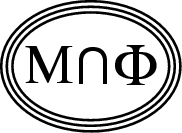Back to
OP page

Combined
List

List by
Contributors

# Soft phases in two-dimensional O(N) models.

### Abstract   Do the spin correlations in the classical Heisenberg model, and other O(N) models with N>2, decay exponentially at all non-zero temperatures, or do the models have soft phases akin to that of the XY model?

It is generally believed by the statistical mechanics and quantum field communities that, unlike the xy-model, the classical Heisenberg O(N) model has exponentially decaying correlations at all temperatures. This belief is based mostly on formal perturbation theory (= low temperature expansion) [1,2]. While for the xy model it has been shown by Bricmont et al  that this expansion is indeed the correct asymptotic expansion in the thermodynamic limit, a proof for the classical Heisenberg model does not exist.

The conventional picture has been challenged by us and we have proposed that the model (and indeed all O(N) models) has a transition to a soft phase with algebraic decay of correlations at low temperature. We gave arguments based on percolation theory  and on the structure of low-lying excitations (super-instantons) ; we discussed the weaknesses of the conventional arguments in .

The problem is of great significance for particle physics, because the classical Heisenberg model is a toy version of QCD, the theory of strong interactions. Existence of a soft phase also in QCD would most likely imply failure of the so-called asymptotic freedom.

1. Prove or disprove that the conventional perturbative expansion (low temperature expansion) gives the correct asymptotic expansion for correlation functions and thermodynamic quantities .

2. Prove or disprove that the model has a critical point at some nonzero temperature.

3. If yes, determine the critical indices; in particular determine if they are temperature dependent like in the xy model.